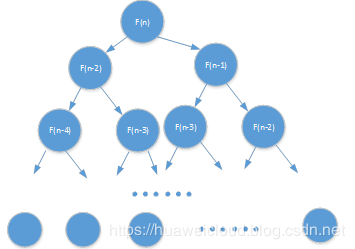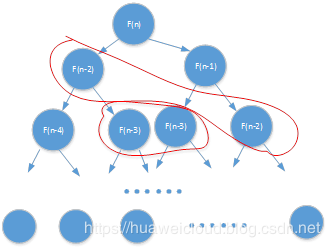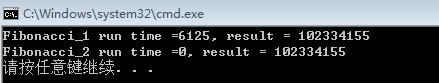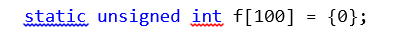# 理解递归与动态规划## 1、从 Fibonacci 函数的四种实现聊起。

Fibonacci 数列，中文也译作斐波那契数列，相信大多数同学不会陌生，就是经典的兔子问题，以下图片内容来源于网络。Fibonacci 数列实现方法 1------- 递归unsigned int Fibonacci_1(unsigned int n) {        if ((n == 1) || (n == 2)) {               return 1;        }          return Fibonacci_1(n - 1) + Fibonacci_1(n - 2); }Fibonacci 数列实现方法 2------- 递归 + 去重复计算

unsigned int Fibonacci_2(unsigned int n) {        static unsigned int f = {0};               if ((n == 1) || (n == 2)) {               return 1;        }        else if (0 != f[n]) {               return f[n];        }          f[n] = Fibonacci_2(n - 1) + Fibonacci_2(n - 2);        return f[n]; }

void testF(void) {        long t1, t2;        unsigned int fn;          t1 = clock();        fn = Fibonacci_1(40);        t2 = clock();          if (t1 <= t2) {               printf("Fibonacci_1 run time =%u, result = %u \n", t2 - t1, fn);        }          t1 = clock();        fn = Fibonacci_2(40);        t2 = clock();          if (t1 <= t2) {               printf("Fibonacci_2 run time =%u, result = %u \n", t2 - t1, fn);        } }Fibonacci 数列实现方法 3------- 正向计算

BTW：顺便提一句，事实上对于 Fibonacci 数列，是有通项公项可以直接计算的，这是高中奥数的基本功。

è C1=1/(5)^(1/2) C2= -1/(5)^(1/2)，得通项公式
èF(n) = ，非本文重点介绍内容，故在此不作过多介绍，如有兴趣，可以私聊。

Fibonacci 数列实现方法 4------ 不动点通项公式

unsigned int Fibonacci_4(unsigned int n) {        double sqrt5 = sqrt(5);        double root1 = (1 + sqrt5) / 2;        double root2 = (1 - sqrt5) / 2;               return (pow(root1, n) - pow(root2, n)) / sqrt5; }

## 3、实战演练。

N=a+a+a+…+a[m];
a[i]>0,1<=m<=N;

4 = 4;
4 = 3 + 1;
4 = 2 + 2;
4 = 2 + 1 + 1;
4 = 1 + 1 + 1 + 1;

4
10
20

5
42
627

## 评论

• ###### Swift 烧脑体操（四） - map 和 flatMap

Swift 其实比 Objective-C 复杂很多，相对于出生于上世纪 80 年代的 Objective-C 来说，Swift 融入了大量新特性。这也使得我们学习掌握这门语言变得相对来说更加困难。不过一切都是值得的，Swift 相比 Objective-C，写出来的程序更安全、更简洁，最终能够提高我们的工作效率和质量。

2016 年 3 月 3 日

• ###### 动态规划理论：一篇文章带你彻底搞懂最优子结构、无后效性和重复子问题

什么样的问题可以用动态规划解决？解决动态规划问题的一般思考过程是什么样的？

2018 年 12 月 31 日

• ###### 递归树：如何借助树来求解递归算法的时间复杂度？

今天我们用递归树分析递归代码的时间复杂度。

2018 年 11 月 21 日

• ###### 初识动态规划：如何巧妙解决“双十一”购物时的凑单问题？

动态规划很不好理解，但是它不难。为了让你更容易理解，我分成三节来讲，分别是：初识动态规划、动态规划理论、动态规划实战。

2018 年 12 月 26 日

• ###### 深度学习中的微分

本文通过自动微分了解程序是如何进行反向传播。

2019 年 11 月 10 日

• ###### 解线性方程组：为什么用矩阵求解的效率这么高？

有弄清楚线性代数这些基础知识的本质，你才能更进一步，去了解其他计算方法。

2020 年 8 月 5 日